Posted on

# Tracking moving objectThis example shows how to separate and track moving object using OpenCV. First, the background of the video is being calculated and moving objects detected, then it is filtered and tracked.

Used: cv::BackgroundSubtractorMOG2; cv::getStructuringElement; cv::morphologyEx; cv::BackgroundSubtractorMOG2.operator();

## The process

1. Initialize the background extraction object
```BackgroundSubtractorMOG2 bg( 500, 64, false);
```
2. Process video frame by background extraction object by it’s method operator and receive mask of moving object
```bg.operator()( origi, mask);
```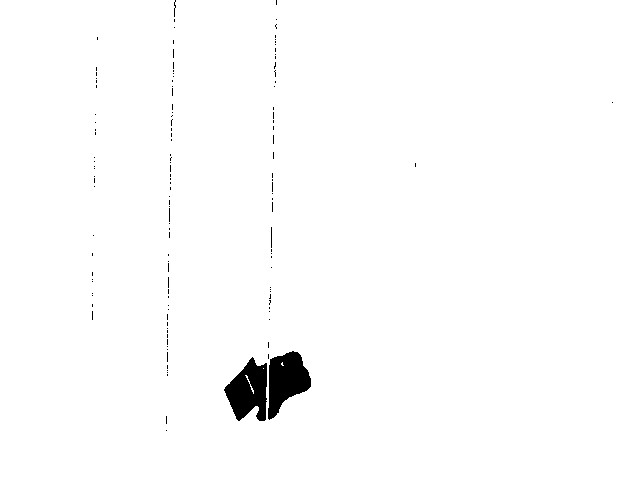```morphologyEx( mask, mask, MORPH_OPEN, element1 );
```
```morphologyEx( mask, mask, MORPH_CLOSE, element2 );
```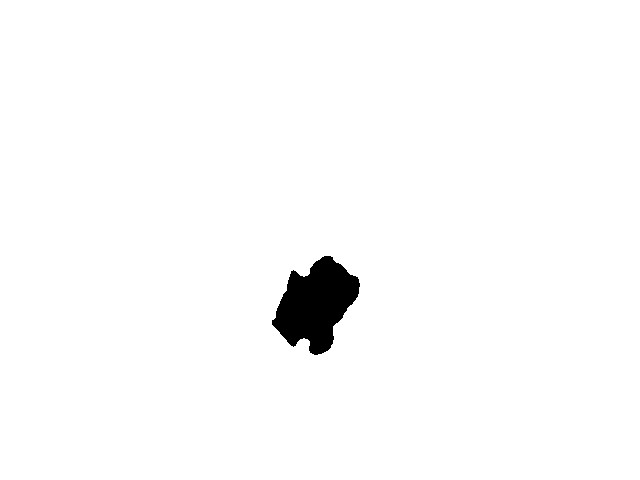5. Apply mask on video frame
```origi.copyTo( proci0, mask);
```
6. Find good features to track and apply KLTracker
```goodFeaturesToTrack( proci0, points, MAX_COUNT, 0.1, 10, Mat(), 3, 0, 0.04);
calcOpticalFlowPyrLK( proci1, proci0, points, points, status, err, winSize, 3, termcrit, 0, 0.00001);
```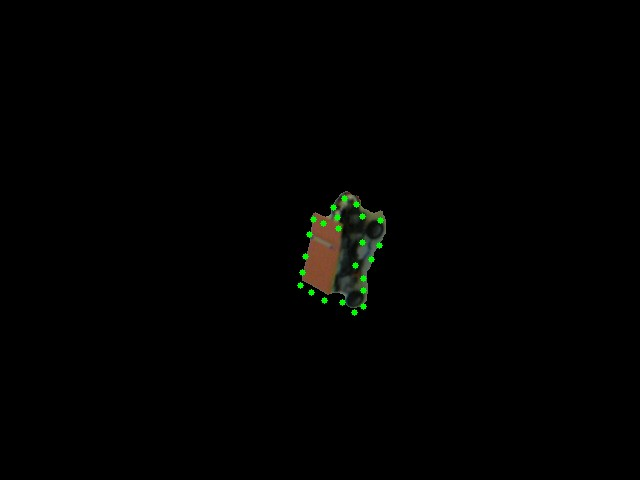7. Combine with initial frame
```size_t i, k;
for (i = k = 0; i &lt; points.size(); i++)
{
if (!status[i]) continue;
points[k++] = points[i];
circle(finI, points[i], 3, Scalar(0, 255, 0), -1, 8);
circle(procI2, points[i], 3, Scalar(0, 255, 0), -1, 8);
}
points.resize(k);
```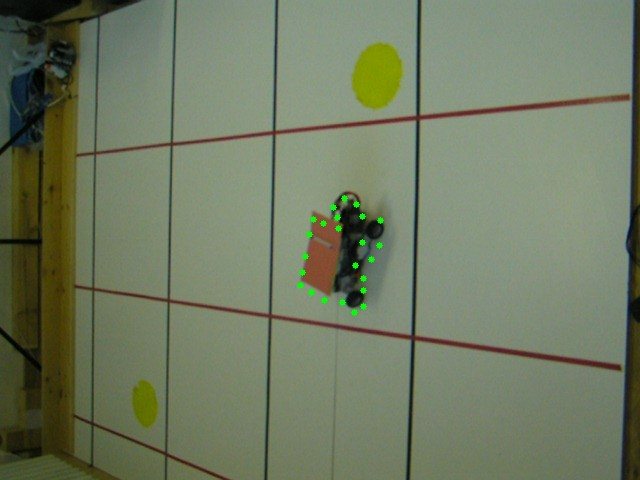8. Find contours of the mask, find it’s bounding rectangle and draw it onto output frame
```findContours(procI3, contours, CV_RETR_LIST, CV_CHAIN_APPROX_SIMPLE);
if (contours.size() &gt; 0)
for (int i = 0; i &lt; (int)contours.size(); i++)
rectangle(finI, boundingRect(contours[i]), Scalar(0, 255, 0));
```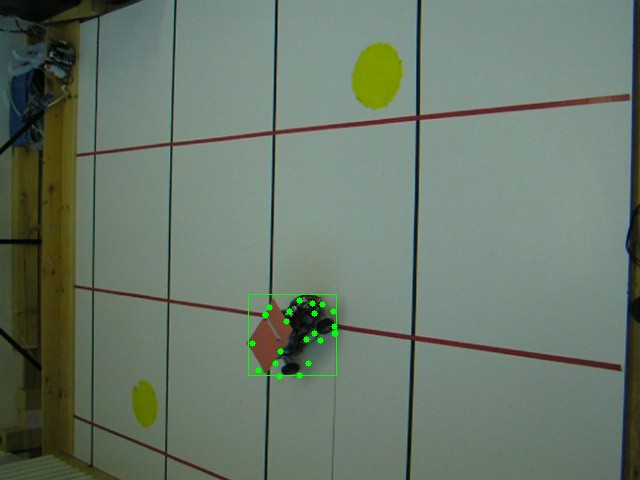Bounding rectangle hints position of moving object on the scene and could be used to approximate it’s coordinates